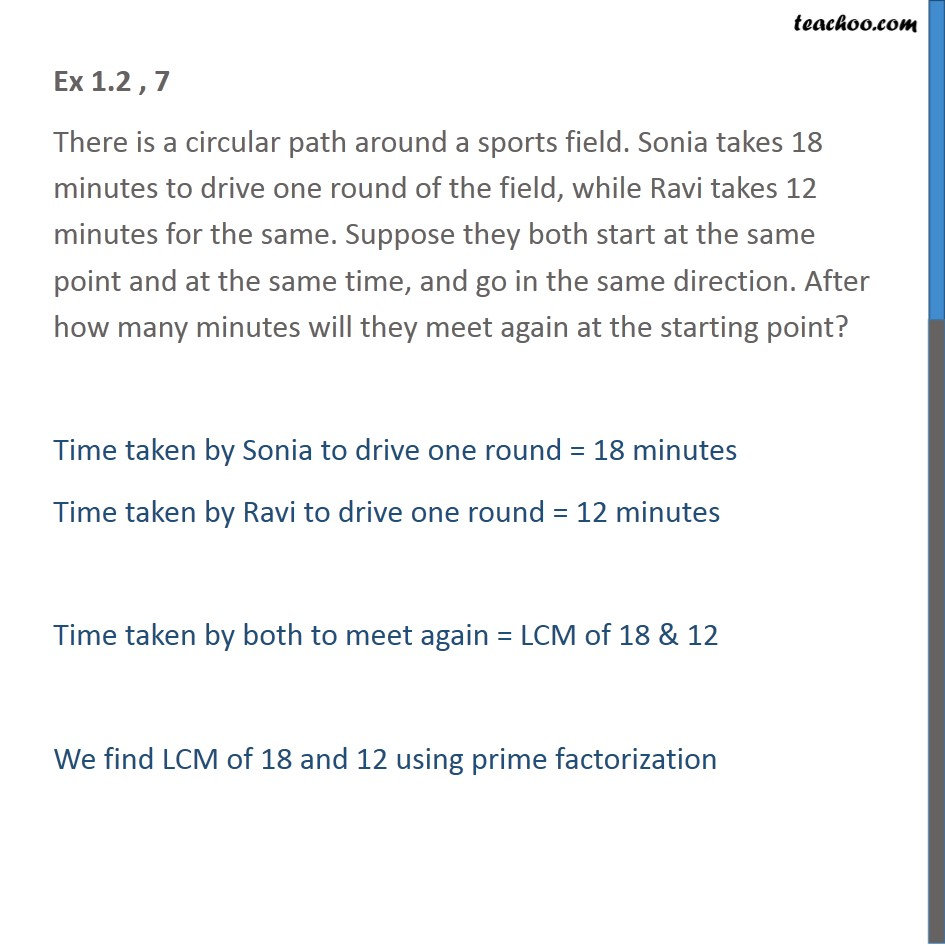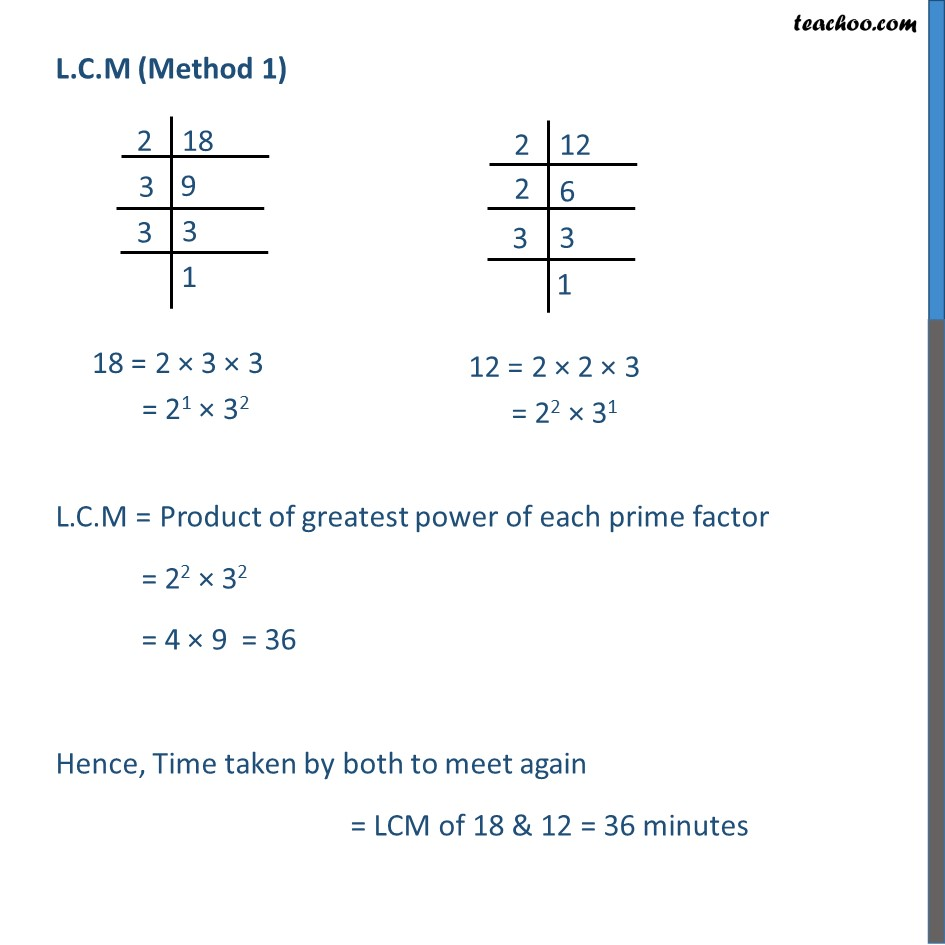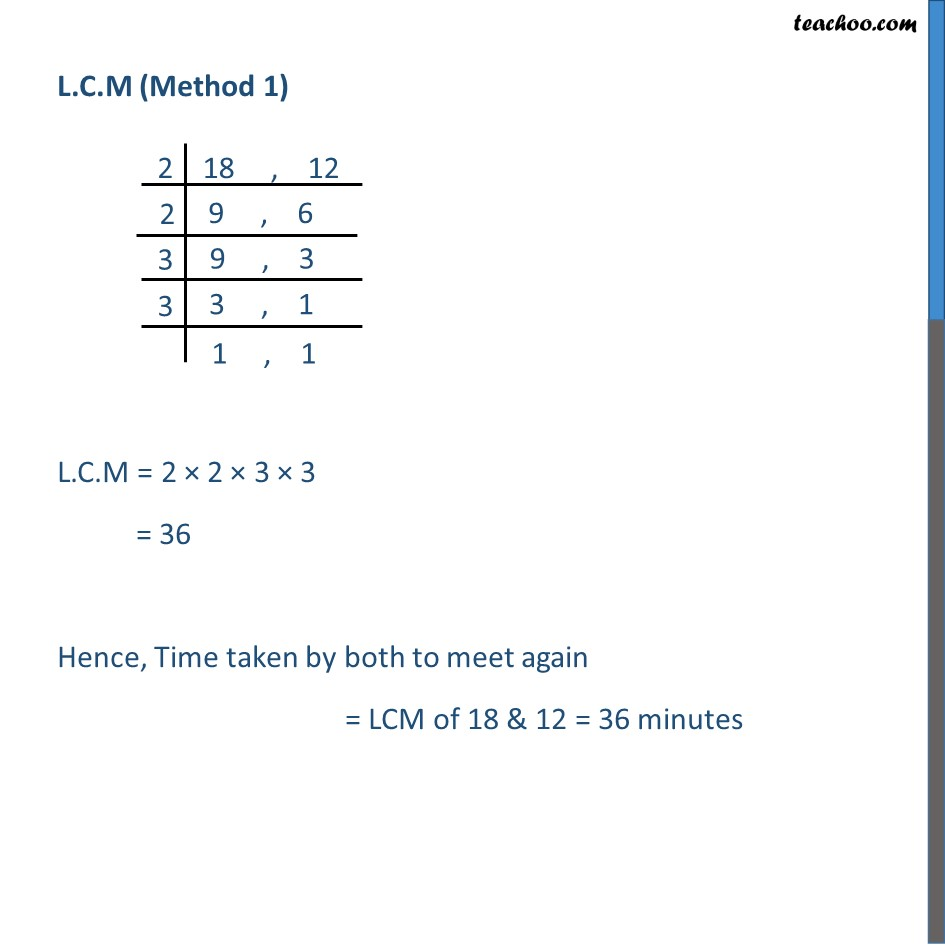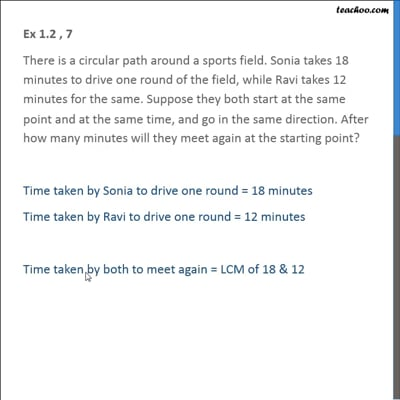Ex 1.2

Chapter 1 Class 10 Real Numbers (Term 1)
Serial order wiseIn video, the answer should be 36 not 54This video is only available for Teachoo black users

### Transcript

Ex 1.2 , 7 There is a circular path around a sports field. Sonia takes 18 minutes to drive one round of the field, while Ravi takes 12 minutes for the same. Suppose they both start at the same point and at the same time, and go in the same direction. After how many minutes will they meet again at the starting point? Time taken by Sonia to drive one round = 18 minutes Time taken by Ravi to drive one round = 12 minutes Time taken by both to meet again = LCM of 18 & 12 We find LCM of 18 and 12 using prime factorization L.C.M (Method 1) L.C.M = Product of greatest power of each prime factor = 22 × 32 = 4 × 9 = 36 Hence, Time taken by both to meet again = LCM of 18 & 12 = 36 minutes L.C.M (Method 1) L.C.M = 2 × 2 × 3 × 3 = 36 Hence, Time taken by both to meet again = LCM of 18 & 12 = 36 minutes